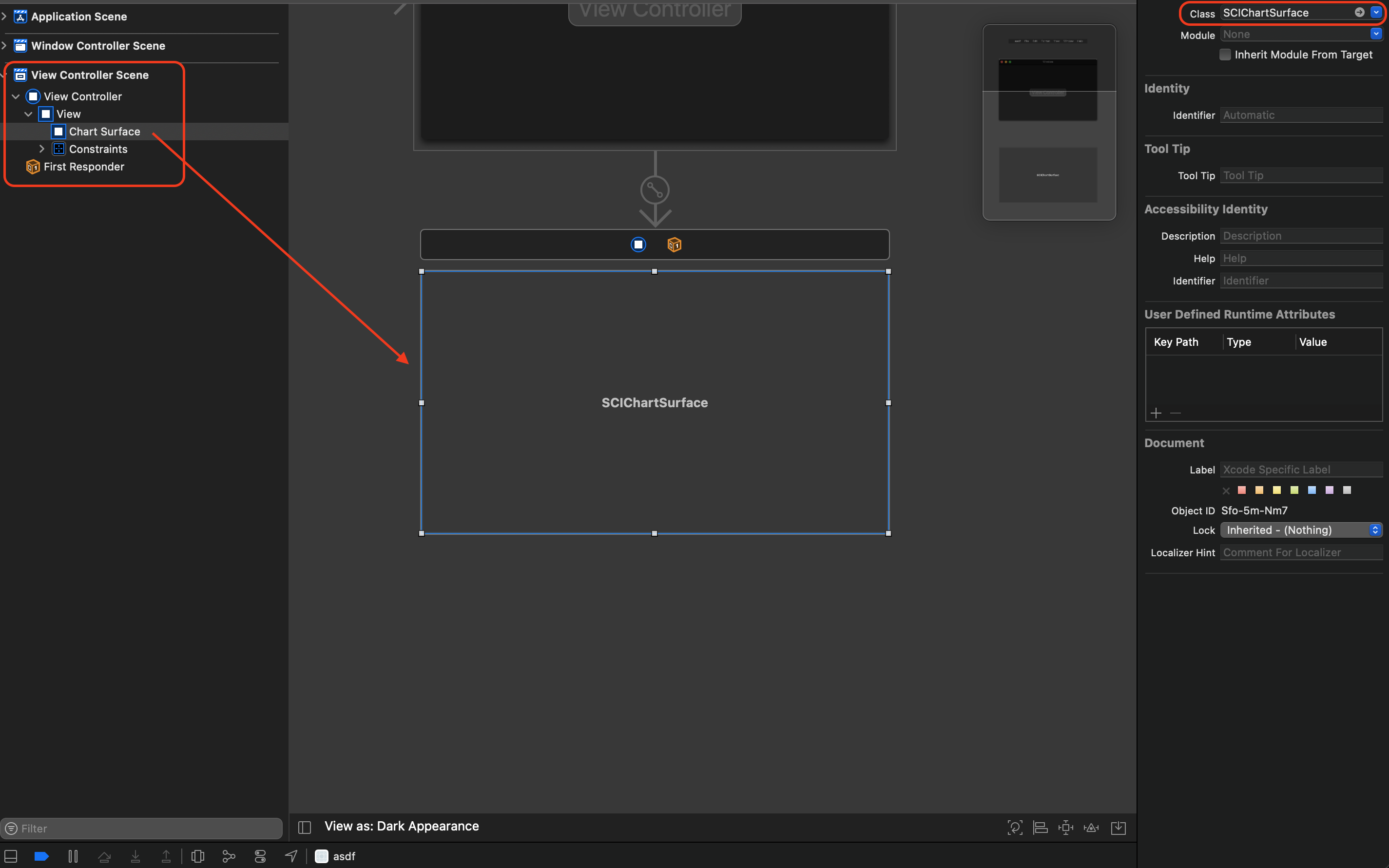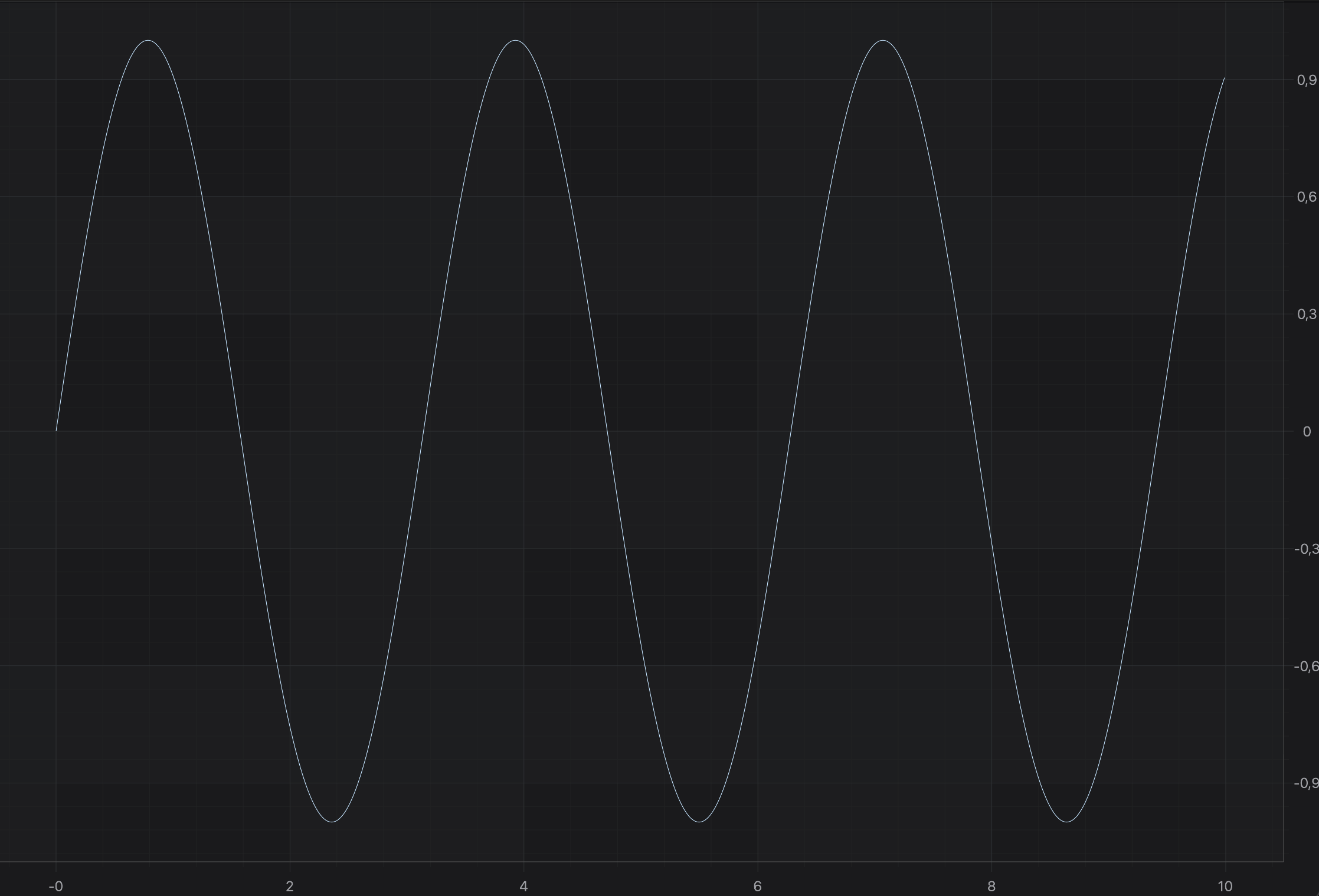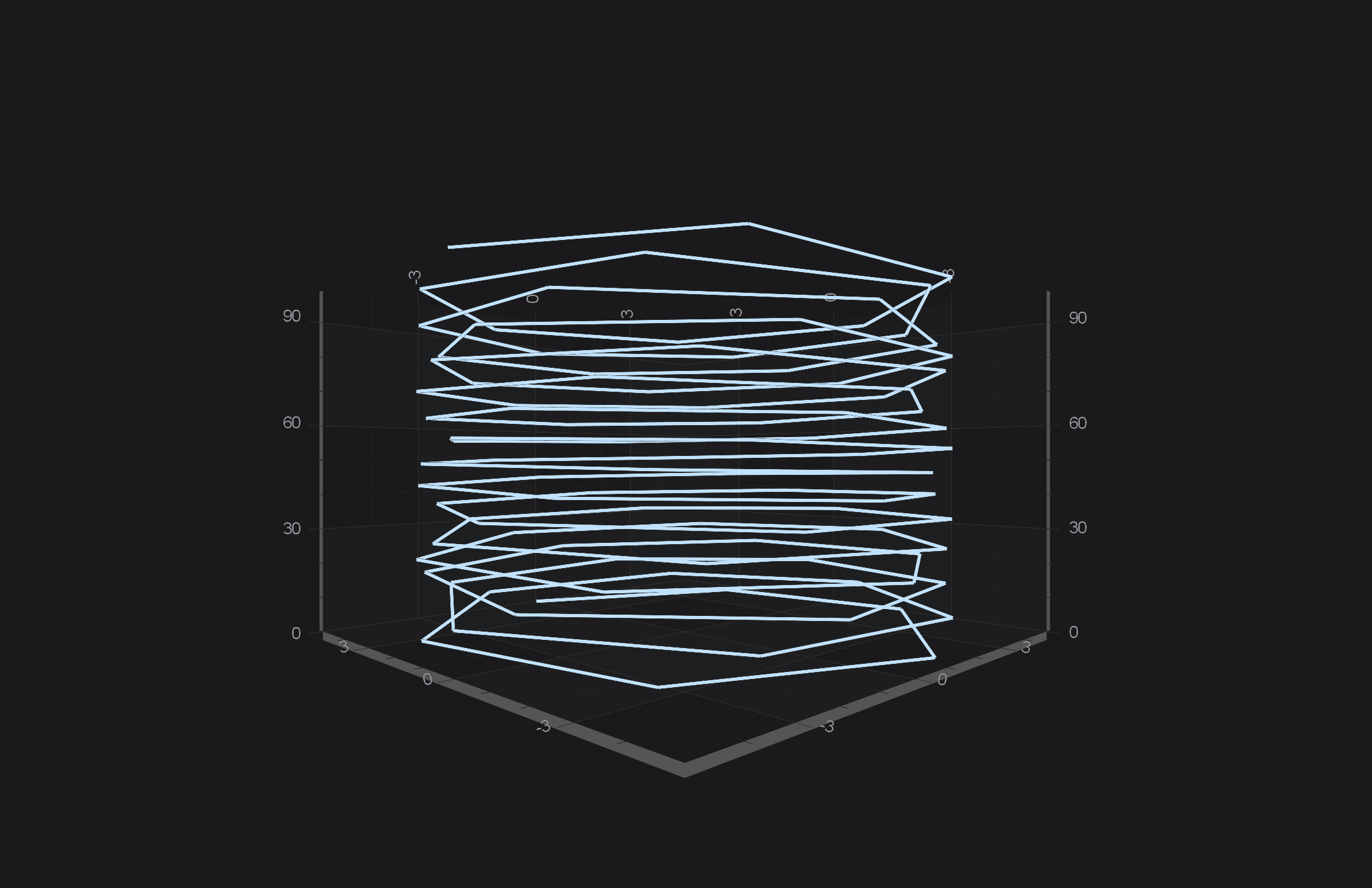iOS & macOS Charting Documentation - SciChart iOS & macOS Charts SDK v4.x

# Creating your first SciChart macOS App

Playing around with SciChart macOS Examples Suite is great, but you are probably interested in creating your own application by adding some charts! Let’s get started and create our first SciChart macOS App using Objective C.

## Setup XCode project

Assuming you’ve prepared development environment and created an App in either `Objective-C` or `Swift` - next step should be integrating SciChart.xcframework. The easiest way of doing so is by using CocoaPods. Add the following to your Podfile, and then run `pod install`

``````source 'https://github.com/ABTSoftware/PodSpecs.git'
platform :osx, '10.13'

use_frameworks!
target 'YourTargetName' do
# Use the latest available Version
pod 'SciChart'
end
``````

NOTE: You can also get the extensive sample app downloading the SciChart macOS Trial package, which can be used for manual integration of SciChart.xcframework.

Before you build and run the application, you will need to apply a trial or purchased license key. You can find full instructions on the page Licensing SciChart.

You can fetch a Trial License Key directly from the Downloads page following instructions from Licensing SciChart macOS. Or, if you have purchased SciChart macOS, you can find the full purchased license keys at your SciChart Account Page.

When you have your key, you should apply it via `+[SCIChartSurface setRuntimeLicenseKey:]` like below:

NOTE: The License Key must be set in your app once, and once only before any `SCIChartSurface` instance is initialized.

From here, you can create 2D or 3D chart. Please refer to the following sections for more information:

## The SCIChartSurface Type

The root 2D chart view is called the `SCIChartSurface`. This is the AppKit NSView you will be adding to your applications wherever you need a chart. You can add more than one `SCIChartSurface`, you can configure them independently, and you can link them together.

Since this is a Quick Start Example, we will use the one instance of `SCIChartSurface`, so let’s start by declaring one!

#### Declaring a SciChartSurface Instance

There few ways of adding `SCIChartSurface` to your application. We will look more closely into the following:

#### Adding SCIChartSurface via the .storyboard

Open up our `.storyboard` file. Add CustomView onto the ViewController and set it’s class to the `SCIChartSurface`. Then add an IBOutlet for your SCIChartSurface in your ViewController code to be able to manipulate with it later on.#### Adding SCIChartSurface purely from code

In your ViewController you will need to import `SciChart` and instantiate the `SCIChartSurface`. See the code below:

#import <SciChart/SciChart.h> … - (void)viewDidLoad { [super viewDidLoad]; SCIChartSurface *surface = [SCIChartSurface new]; surface.translatesAutoresizingMaskIntoConstraints = NO; [self.view addSubview:surface]; [surface.topAnchor constraintEqualToAnchor:self.view.safeAreaLayoutGuide.topAnchor].active = YES; [surface.bottomAnchor constraintEqualToAnchor:self.view.safeAreaLayoutGuide.bottomAnchor].active = YES; [surface.leftAnchor constraintEqualToAnchor:self.view.leftAnchor].active = YES; [surface.rightAnchor constraintEqualToAnchor:self.view.rightAnchor].active = YES; }
import SciChart … override func viewDidLoad() { super.viewDidLoad() let surface = SCIChartSurface() surface.translatesAutoresizingMaskIntoConstraints = false self.view.addSubview(surface) surface.topAnchor.constraint(equalTo: self.view.safeAreaLayoutGuide.topAnchor).isActive = true surface.bottomAnchor.constraint(equalTo: self.view.safeAreaLayoutGuide.bottomAnchor).isActive = true surface.leftAnchor.constraint(equalTo: self.view.leftAnchor).isActive = true surface.rightAnchor.constraint(equalTo: self.view.rightAnchor).isActive = true }

#### Adding Axes to the SCIChartSurface

Once you have added a `SCIChartSurface` into your ViewController, you will not see anything drawn because you need to add axes. This is an important thing here - two axes X and Y has to be added to your surface. This is a bare minimum to see a drawn grid on your device.

Now, we would like to see something more than just an empty grid, e.g. Line Chart. So let’s add some RenderableSeries with appropriate DataSeries to our surface:

const int count = 1000; SCIDoubleValues *xValues = [[SCIDoubleValues alloc] initWithCapacity:count]; SCIDoubleValues *yValues = [[SCIDoubleValues alloc] initWithCapacity:count]; for (int i = 0; i < count; i++) { double x = 10.0 * i / count; double y = sin(2 * x); [xValues add:x]; [yValues add:y]; } id<ISCIXyDataSeries> dataSeries = [[SCIXyDataSeries alloc] initWithXType:SCIDataType_Double yType:SCIDataType_Double]; [dataSeries appendValuesX:xValues y:yValues]; id<ISCIRenderableSeries> rSeries = [SCIFastLineRenderableSeries new]; rSeries.dataSeries = dataSeries; [self.surface.renderableSeries add:rSeries];
let count = 1000 let xValues = SCIDoubleValues(capacity: count) let yValues = SCIDoubleValues(capacity: count) for i in 0 ..< count { let x: Double = 10.0 * Double(i) / Double(count) let y: Double = sin(2 * x) xValues.add(x) yValues.add(y) } let dataSeries = SCIXyDataSeries(xType: .double, yType: .double) dataSeries.append(x: xValues, y: yValues) let renderableSeries = SCIFastLineRenderableSeries() renderableSeries.dataSeries = dataSeries self.surface.renderableSeries.add(renderableSeries)

NOTE: You might have noticed, that we used `SCIDoubleValues` while appending points to `ISCIXyDataSeries`. That’s the recommended way of appending data, due to better performance, comparing to adding points one by one. You can use `-[ISCIXyDataSeries appendX:y:]` if you want though.

#### Final example code

So let’s see what we’ve managed to get. Let’s see the listing from the ViewController below:

#import “ViewController.h” #import <SciChart/SciChart.h> @interface ViewController () // Surface is added in Storyboard @property (weak, nonatomic) IBOutlet SCIChartSurface *surface; @end @implementation ViewController - (void)viewDidLoad { [super viewDidLoad]; id<ISCIAxis> xAxis = [SCINumericAxis new]; xAxis.growBy = [[SCIDoubleRange alloc] initWithMin:0.05 max:0.05]; id<ISCIAxis> yAxis = [SCINumericAxis new]; yAxis.growBy = [[SCIDoubleRange alloc] initWithMin:0.05 max:0.05]; const int count = 1000; SCIDoubleValues *xValues = [[SCIDoubleValues alloc] initWithCapacity:count]; SCIDoubleValues *yValues = [[SCIDoubleValues alloc] initWithCapacity:count]; for (int i = 0; i < count; i++) { double x = 10.0 * i / count; double y = sin(2 * x); [xValues add:x]; [yValues add:y]; } id<ISCIXyDataSeries> dataSeries = [[SCIXyDataSeries alloc] initWithXType:SCIDataType_Double yType:SCIDataType_Double]; [dataSeries appendValuesX:xValues y:yValues]; id<ISCIRenderableSeries> rSeries = [SCIFastLineRenderableSeries new]; rSeries.dataSeries = dataSeries; [SCIUpdateSuspender usingWithSuspendable:self.surface withBlock:^{ [self.surface.xAxes add:xAxis]; [self.surface.yAxes add:yAxis]; [self.surface.renderableSeries add:rSeries]; }]; } @end
import UIKit import SciChart class ViewController: UIViewController { // Surface is added in Storyboard @IBOutlet weak var surface: SCIChartSurface! override func viewDidLoad() { super.viewDidLoad() let xAxis = SCINumericAxis() xAxis.growBy = SCIDoubleRange(min: 0.05, max: 0.05) let yAxis = SCINumericAxis() yAxis.growBy = SCIDoubleRange(min: 0.05, max: 0.05) let count = 1000 let xValues = SCIDoubleValues(capacity: count) let yValues = SCIDoubleValues(capacity: count) for i in 0 ..< count { let x: Double = 10.0 * Double(i) / Double(count) let y: Double = sin(2 * x) xValues.add(x) yValues.add(y) } let dataSeries = SCIXyDataSeries(xType: .double, yType: .double) dataSeries.append(x: xValues, y: yValues) let renderableSeries = SCIFastLineRenderableSeries() renderableSeries.dataSeries = dataSeries SCIUpdateSuspender.usingWith(self.surface) { self.surface.xAxes.add(xAxis) self.surface.yAxes.add(yAxis) self.surface.renderableSeries.add(renderableSeries) } } }

NOTE: Please note that we’ve added axes and renderableSeries to `SCIChartSurface` inside `+[SCIUpdateSuspender usingWithSuspendable:withBlock:]` block. This allows you to suspend surface instance, and refresh it only one time after you finished all needed operations. That’s highly recommended technique if you want to omit performance decrease due to triggering refreshes on every operation which could be performed in one batch.## The SCIChartSurface3D Type

The root 3D chart view is called the `SCIChartSurface3D`. This is the AppKit NSView which you will be adding to your applications wherever you need a 3D chart You can add more than one `SCIChartSurface3D`, you can configure them independently and you can link them together.

Since this is a Quick Start Example, we will use the one instance of `SCIChartSurface3D`, so let’s start by declaring one!

#### Declaring a SCIChartSurface3D Instance

Declaring `SCIChartSurface3D` to your application is no different than the regular SCIChartSurface, so please refer to the corresponding section in this article.

#### Adding 3D Axes to the SCIChartSurface3D

Once you have added a `SCIChartSurface3D` into your ViewController, you will not see anything drawn because you need to add axes. This is an important thing here - three axes X, Y, and Z has to be added to your surface. This is a bare minimum to see a drawn grid on your device.

self.surface.xAxis = [SCINumericAxis3D new]; self.surface.yAxis = [SCINumericAxis3D new]; self.surface.zAxis = [SCINumericAxis3D new];
self.surface.xAxis = SCINumericAxis3D() self.surface.yAxis = SCINumericAxis3D() self.surface.zAxis = SCINumericAxis3D()

Now, we would like to see something more than just an empty grid, e.g. Scatter 3D Chart. So let’s add some RenderableSeries3D with appropriate DataSeries 3D to our surface:

SCIXyzDataSeries3D *dataSeries = [[SCIXyzDataSeries3D alloc] initWithXType:SCIDataType_Double yType:SCIDataType_Double zType:SCIDataType_Double]; for (int i = 0; i < 100; ++i) { double x = 5 * sin(i); double y = i; double z = 5 * cos(i); [dataSeries appendX:@(x) y:@(y) z:@(z)]; } SCIPointLineRenderableSeries3D *rSeries = [SCIPointLineRenderableSeries3D new]; rSeries.dataSeries = dataSeries; rSeries.strokeThickness = 3.0; [self.surface.renderableSeries add:rSeries];
let dataSeries = SCIXyzDataSeries3D(xType: .double, yType: .double, zType: .double) for i in 0 ..< 100 { let x = 5 * sin(Double(i)) let y = Double(i) let z = 5 * cos(Double(i)) dataSeries.append(x: x, y: y, z: z) } let rSeries = SCIPointLineRenderableSeries3D() rSeries.dataSeries = dataSeries rSeries.strokeThickness = 3.0 self.surface.renderableSeries.add(rSeries)

#### Final example 3D code

So let’s see what we’ve managed to get. Let’s see the listing from the ViewController below:

#import “ViewController.h” #import <SciChart/SciChart.h> @interface ViewController () // Surface is added in Storyboard @property (weak, nonatomic) IBOutlet SCIChartSurface3D *surface; @end @implementation ViewController - (void)viewDidLoad { [super viewDidLoad]; SCIXyzDataSeries3D *dataSeries = [[SCIXyzDataSeries3D alloc] initWithXType:SCIDataType_Double yType:SCIDataType_Double zType:SCIDataType_Double]; for (int i = 0; i < 100; ++i) { double x = 5 * sin(i); double y = i; double z = 5 * cos(i); [dataSeries appendX:@(x) y:@(y) z:@(z)]; } SCIPointLineRenderableSeries3D *rSeries = [SCIPointLineRenderableSeries3D new]; rSeries.dataSeries = dataSeries; rSeries.strokeThickness = 3.0; [SCIUpdateSuspender usingWithSuspendable:self.surface withBlock:^{ self.surface.xAxis = [SCINumericAxis3D new]; self.surface.yAxis = [SCINumericAxis3D new]; self.surface.zAxis = [SCINumericAxis3D new]; [self.surface.renderableSeries add:rSeries]; }]; } @end
import UIKit import SciChart class ViewController: UIViewController { // Surface is added in Storyboard @IBOutlet weak var surface: SCIChartSurface3D! override func viewDidLoad() { super.viewDidLoad() let dataSeries = SCIXyzDataSeries3D(xType: .double, yType: .double, zType: .double) for i in 0 ..< 100 { let x = 5 * sin(Double(i)) let y = Double(i) let z = 5 * cos(Double(i)) dataSeries.append(x: x, y: y, z: z) } let rSeries = SCIPointLineRenderableSeries3D() rSeries.dataSeries = dataSeries rSeries.strokeThickness = 3.0 SCIUpdateSuspender.usingWith(self.surface) { self.surface.xAxis = SCINumericAxis3D() self.surface.yAxis = SCINumericAxis3D() self.surface.zAxis = SCINumericAxis3D() self.surface.renderableSeries.add(rSeries) } } }

NOTE: Please note that we’ve added axes and renderableSeries to `SCIChartSurface3D` inside `+[SCIUpdateSuspender usingWithSuspendable:withBlock:]` block. This allows you to suspend surface instance, and refresh it only one time after you finished all needed operations. That’s highly recommended technique if you want to omit performance decrease due to triggering refreshes on every operation which could be performed in one batch.